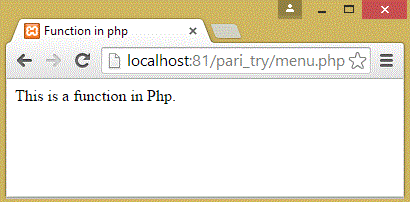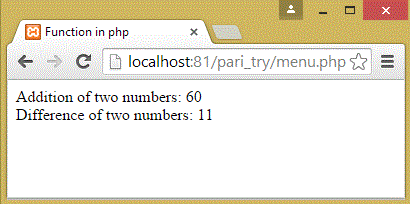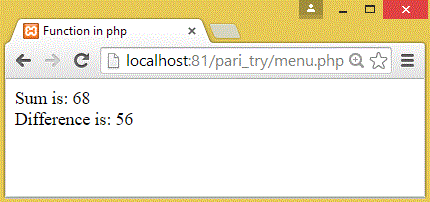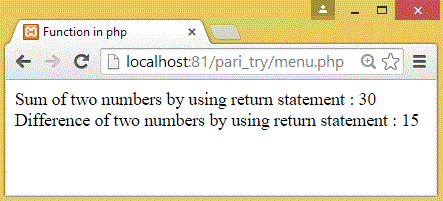# PHP Programing language

Function in PHP
 Previous Home Next

### Functions

A function is a block of statements that can be used repeatedly in a program. It will not execute immediately when a page loads. It will be executed by a call to the function.

A function is a piece of code which takes input in the form of parameter and after processing returns a value. Php functions are similar to other programming language functions. To function declaration, there is a need of keyword ‘function’.

Syntax to declare a function:
```function function_name()
{
block of code to be executed;
}
```

### Example:

```<html>
<head>
<title>Function in php</title>
</head>
<body>
<?php
function func()
{
echo "This is a function in Php.";
}
func();
?>
</body>
</html>
```

Output:Function Argument pass by Value

In php, function pass the value inside it by using name as parameter. Parameter is specified after the function name inside the parentheses. you can pass more than one parameter in the function seperated by commas(,).

Syntax:
```function function_name(\$var1, \$var2,.....\$varN)
{
block of code to be executed;
}
```

### Example:

```<html>
<head>
<title>Function in php</title>
</head>
<body>
<?php
function total(\$num1, \$num2)
{
\$sum = \$num1 + \$num2;
echo "Addition of two numbers: \$sum<br/>";
}
total(23, 37);
function difference(\$num1, \$num2)
{
\$sub = \$num1 - \$num2;
echo "Difference of two numbers: \$sub<br/>";
}
difference(49, 38);
?>
</body>
</html>
```

Output:Function Arguments Pass by Reference

In php, function pass the value by reference inside it by using reference of vaiable. you can pass more than one reference in the function seperated by commas(,). you can pass values by reference, the function receives a reference to the actual values.

Syntax:
```function function_name(&\$var1, &\$var2,.....&\$varN)
{
block of code to be executed;
}
```

### Example:

```<html>
<head>
<title>Function in php</title>
</head>
<body>
<?php
function sum(&\$num)

{
\$num += 10;
}
function difference(&\$num)
{
\$num -= 12;
}
\$var = 58;
sum( \$var );
echo "Sum is: \$var<br/>";
difference(\$var);
echo " Difference is: \$var<br/>";
?>
</body>
</html>
```

Output:Functions retruning value

A function can return a value using the return statement in conjunction with a value or object. You can return more than one value from a function using return array(arr1,arr2,arr3,arr4).

Syntax:
```function function_name(\$var1, \$var2,.....\$varN)
{
block of code to be executed;
reuturn statement;
}
```

### Example:

```<html>
<head>
<title>Function in php</title>
</head>
<body>
<?php
function total(\$num1, \$num2)
{
\$sum = \$num1 + \$num2;
return \$sum;
}
function sub(\$num1, \$num2)
{
\$diff = \$num1 - \$num2;
return \$diff;
}
\$return_value = total(10, 20);
echo "Sum of two numbers by using return statement : \$return_value<br/>";
\$return_value = sub(38, 23);
echo "Difference of two numbers by using return statement
: \$return_value";
?>
</body>
</html>
```

Output:Previous Home Next